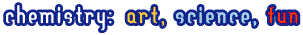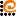### Problems### Best tasks from the past

In this section we publish best tasks from the previous IChO in Moscow held in 1996. Solution follows text of the problem with a two weeks gap. The tasks will be changed once a month. Have a nice time!The Best IChO tasks from 1980-1990Word

## Moscow, 1996. Inorganic chemistry

Potassium dichromate is one of the most widely used precipitating reagents. The following equilibria are established in aqueous solutions of Cr(VI).
HCrO4- + H2O = CrO42- + H3O+ pK1 = 6.50
2HCrO4- = Cr2O72- + H2O pK2 = -1.36
All other equlibria with involving of chromium should be ignored. All the activity coefficients are assumed to be 1.
1. The ionic product of water Kw = 1.0∙10-14. Evaluate the equilibrium constants
a) CrO42- + H2O = HCrO4- + OH-
b) Cr2O72- + 2OH- = 2CrO42- + H2O
2. The solubility product of BaCrO4 Ks = 1.2∙10-10. BaCr2O7 is very soluble in water. In what direction will the equilibrium 1b shift upon the addition of the following reagents to a moderately concentrated aqueous solution of potassium dichromate?
a) KOH
b) HCl
c) BaCl2
d) H2O (consider all the above equilibria).
3. Dissociation constant of acetic acid Ka = 1.8∙10-5. Calculate the pH value of the following solutions
a) 0.010 M K2CrO4
b) 0.010 M K2Cr2O7
c) 0.010 M K2Cr2O7 + 0.10 M CH3COOH
4. Calculate the equilibrium concentrations of the following ions in a solution of 0.010 M K2Cr2O7 + 0.10 M CH3COOH
a) CrO42-
b) Cr2O72-

## Moscow, 1996. Physical chemistryMoscow, 1996. Organic chemistry

| ©2006-2007 Chemisty Department of Moscow State University |© web-design www.AdCode.ru